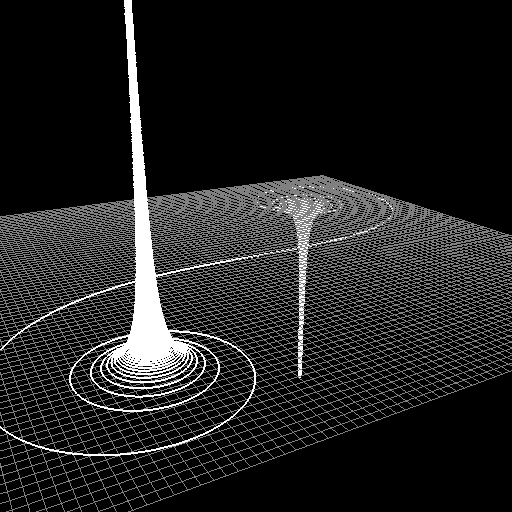$\newcommand{\sech}{\mathop{\rm sech}\nolimits} \newcommand{\csch}{\mathop{\rm csch}\nolimits} \newcommand{\R}{\mathbb{R}} \newcommand{\basis}{\underline{\mathbf{e}}}$
Current Page
Euler spiral

# Euler spiral

Object type: Plane curve

### Definition

In $\mathbb{R}^2$, an Euler spiral (sometimes a Cornu spiral) is a curve, the signed curvature of which is proportional to the arc length measured from some fixed point on the curve.### Parameterisation

The Euler spiral $\mathbf{r}(\mathbb{R})$ where $$\mathbf{r}(t) = \left(\sqrt{\frac {2}{a}}C\left(\sqrt{\frac{a}{2}}t\right), \sqrt{\frac{2}{a}}S\left(\sqrt{\frac{a}{2}}t \right)\right)$$where the Fresnel integrals are $$C(x) := \int_0^x {\cos{t^2}dt}, \quad\quad S(x) := \int_0^x {\sin{t^2}dt}$$ has curvature function $$\kappa(t) = a t$$ and $t$ is the arc-length parameter. Above the part $\mathbf{r}\left(\left[-10, 10 \right]\right)$ is shown for $a = 2$.

### Examples

Needless to say, Euler spirals are used in road and railway construction. In addition, the Euler spiral is studied in diffraction theory.

### Trivia

It is tempting to make a space curve where the $z$ coordinate is proportional to arc length and the projection of the curve onto the $xy$ plane is an Euler spiral. One such space curve is shown below.